# SPTK: Examples of Random Variables in Communication-Signal Contexts

Some examples of random variables encountered in communication systems, channels, and mathematical models.

Previous SPTK Post: Random Variables Next SPTK Post: Random Processes

In this Signal Processing ToolKit post, we continue our exploration of random variables. Here we look at specific examples of random variables, which means that we focus on concrete well-defined cumulative distribution functions (CDFs) and probability density functions (PDFs). Along the way, we show how to use some of MATLAB’s many random-number generators, which are functions that produce one or more instances of a random variable with a specified PDF.

### Common Random Variables

#### Uniform

This random variable is constrained to take values on some finite interval. The distribution is ‘uniform’ because the probability that the variable takes on values in any subinterval of length$\Delta$ is independent of where that subinterval lies-no subinterval is more or less probable than any other.

We looked at the uniform random variable in some detail in the introductory post on random variables. In communication-signal contexts, it is commonly used to model parameters of a received signal for which no significant prior information is available. A good example is carrier phase. The phase of the sine-wave carrier used in an RF communication signal is not known to the receiver, but we have no way of favoring one set of phase values over any other, so we simply say it is uniformly distributed on some interval with width$2\pi$, which covers all possible phases without ambiguity or omission. Typically the interval is$[-\pi, \pi)$ or$[0, 2\pi)$.

MATLAB’s uniform random variable generator is rand.m, which produces one or more instances of the random variable on the interval$(0, 1)$. I generated$100,000$ instances using rand.m and then estimated the PDF using MATLAB’s histcounts.m. Integrating the resulting PDF estimate provides an estimate of the CDF. The CDF and PDF are shown in Figures 1 and 2.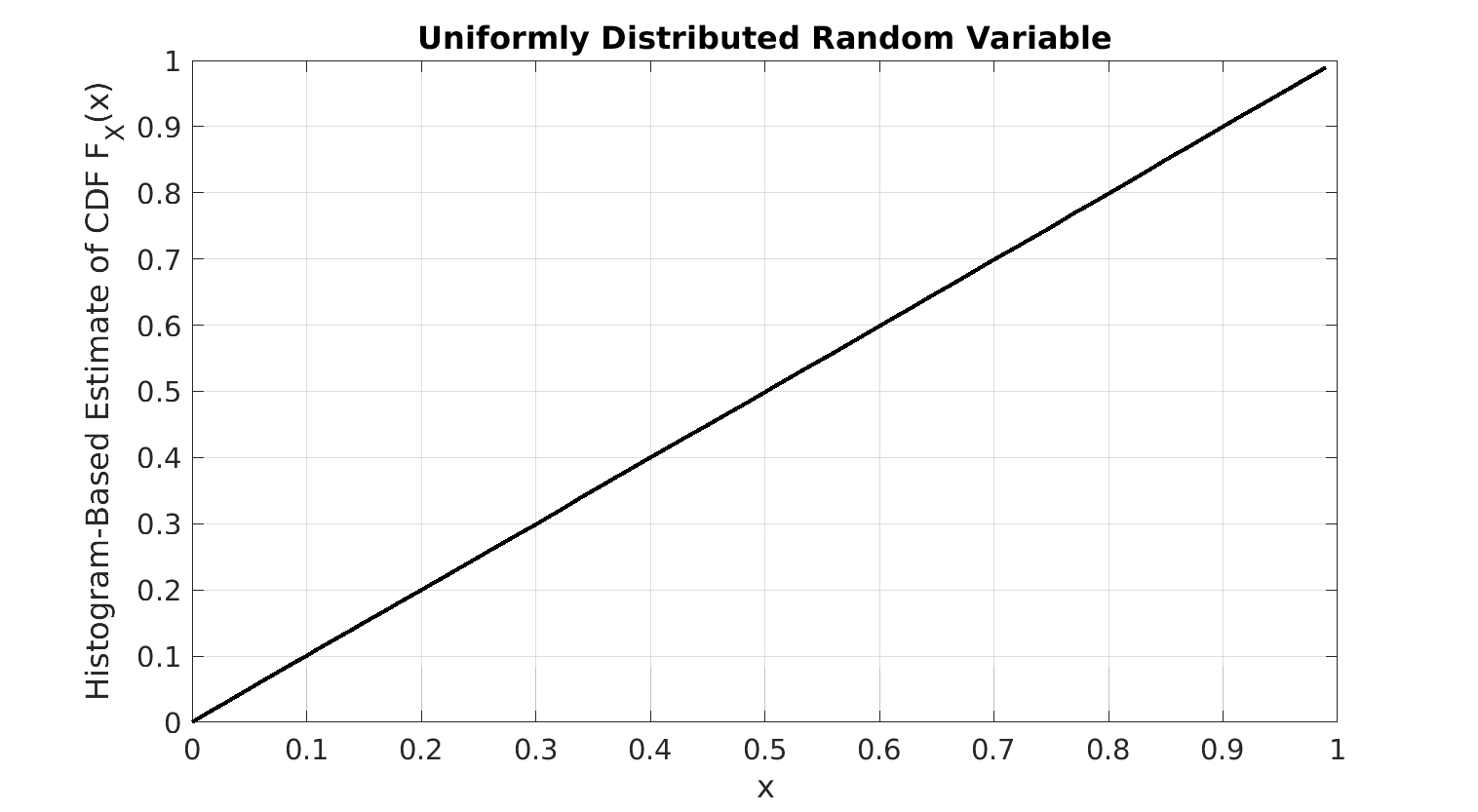Figure 1. Estimated cumulative distribution function for a MATLAB-generated uniform random variable on the interval .

To generate a uniform random variable on some other interval$(A, B)$, multiply the instance returned by rand.m by$B-A$ and add$A$.

You should compare the reported statistics in green in Figure 2 with the formulas for the probabilistic parameters we wrote down in the previous post on random variables.

To use histcounts.m to estimate the probability density function of a collection of numbers (typically they will be instances of some random variable so that the notion of a probability density function applies), I use this kind of function call:

[counts, locs] = histcounts(X, num_bins, ‘normalization’, ‘pdf’);

where X is the vector of numbers, num_bins is an integer that specifies the number of subintervals with which to divide up the x-axis, and the type of normalization applied to the histogram values corresponds to a PDF (other normalizations are offered as well). The counts of the values in X that land in each of the num_bins bins along the x-axis are returned in counts, and the edges of the histcounts.m-determined bins are returned in locs. I can then simply plot the counts against the locs.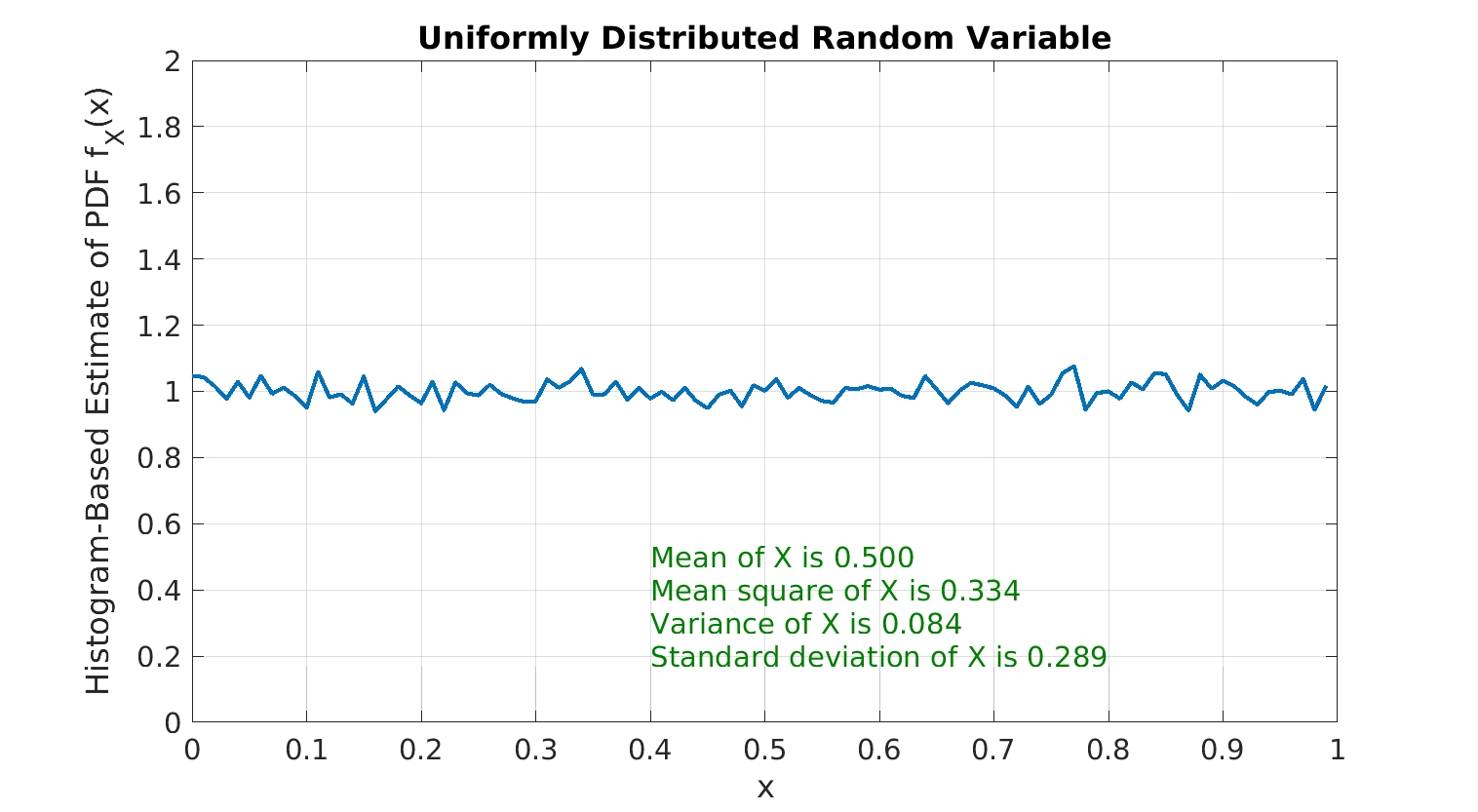Figure 2. Estimated probability density function for a MATLAB-generated uniform random variable on the interval .

#### Gaussian (Normal)

The Gaussian, or normal, random variable is the most common random variable used in communication-system theory, design, and practice. MATLAB’s Gaussian random number generator is randn.m. Using randn.m and histcounts.m, I estimated the CDF and PDF as shown in Figures 3 and 4. randn.m produces instances that conform to a Gaussian distribution with mean of zero and variance of one (and therefore the standard deviation is also one). To obtain other means, add the desired mean to the instance. To obtain other variances, multiply the instance by the desired standard deviation.Figure 3. Estimated cumulative distribution function for a MATLAB-generated Gaussian random variable with mean of zero and variance of one.

Note that the reported statistics in Figure 4 are very close to the randn.m mean and variance values of zero and one.Figure 4. Estimated probability density function for a MATLAB-generated Gaussian random variable with mean of zero and variance of one.

Gaussian random variables are universally used to model the thermal noise we experience in our RF-communication reception equipment. In particular, such noise is modeled as white (impulsive autocorrelation, constant power spectrum) and Gaussian, and it simply adds to the values of the impinging electromagnetic wave: Additive White Gaussian Noise (AWGN).

#### Squared Gaussian (Squared Normal)

The squared-normal random variable is just the square of a Gaussian random variable. This kind of variable can arise when one is looking at the statistics, for example, of the power or energy of a Gaussian random variable. To form a squared-normal random variable, I simply generate a zero-mean unit-variance Gaussian using randn.m and square it. The estimated cumulative distribution function and probability density function for the squared Gaussian variable are shown in Figures 5 and 6.Figure 5. Estimated cumulative distribution function for a MATLAB-generated squared Gaussian random variable. The underlying Gaussian random variable has mean of zero and variance of one.Figure 6. Estimated probability density function for a MATLAB-generated squared Gaussian random variable. The underlying Gaussian random variable has mean of zero and variance of one.

The squared Gaussian random variable is a special case of the chi-squared distribution (see below). A chi-squared random variable is the sum of$N$ squared Gaussian variables, so our squared Gaussian here corresponds to$N=1$. The mean of a chi-squared random variable with parameter$N$ (‘$N$ degrees of freedom’) is$N$ and the variance is$2N$. Compare those predicted values with the statistics reported in Figure 6.

#### Rayleigh

A Rayleigh random variable is the square root of the sum of the squares of two Gaussian random variables,$\displaystyle Z = \sqrt(X^2 + Y^2), \hfill (1)$

where$X$ and$Y$ are zero-mean independent Gaussian random variables with variances$\sigma^2$. If you think of$X$ and$Y$ as components of a two-dimensional vector (coordinates in two-dimension space, say), then the Rayleigh variable is the distance (magnitude) of the vector.

There are at least two ways to generate instances of Rayleigh random variables in MATLAB: using randn.m and using raylrnd.m. To use randn.m, just call it twice to create two independent zero-mean unit-variance Gaussian random variables, and then compute the square root of the sum of their squares. Get different versions of the Rayleigh distribution by modifying the variances of the obtained Gaussian variables prior to the square/square-root computation. Using raylrnd.m is easy-it requires only a single parameter$B$, which is just$\sigma$ here.

I used both methods to estimate the CDF and PDF for a Rayleigh random variable with parameter$B = \sigma = 1$ and plotted the results in Figures 7 and 8.Figure 7. Estimated cumulative distribution function for a Rayleigh random variable with parameter done two ways: with randn.m and with raylrnd.m.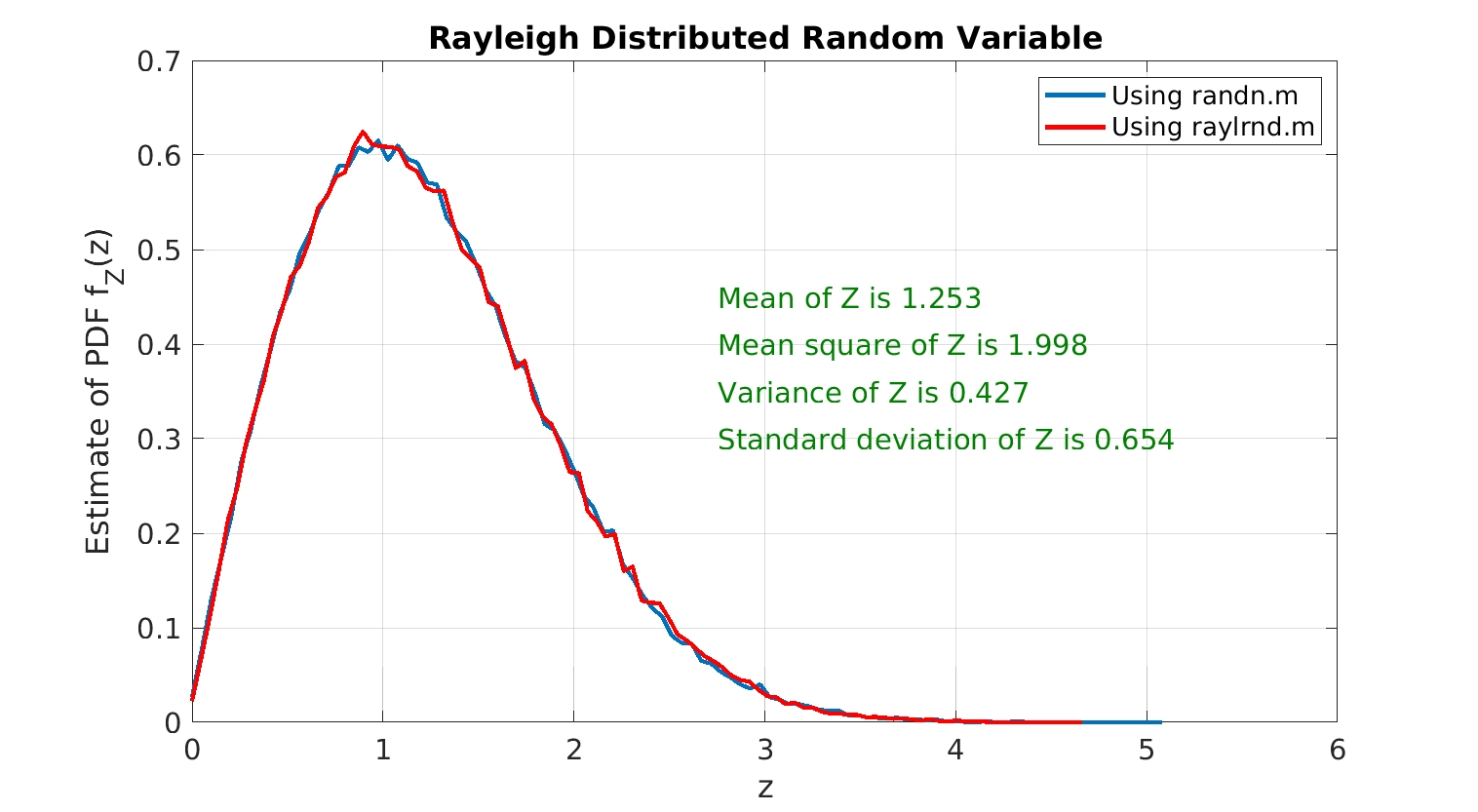Figure 8. Estimated probability density function for a Rayleigh random variable with parameter done two ways: with randn.m and with raylrnd.m.

The mean of the Rayleigh random variable is$\sigma \sqrt(\pi/2)$ and the variance is$\sigma^2(4-\pi)/2$, which for$\sigma^2 = \sigma = B = 1$ are$1.25$ and$0.429$, respectively. Compare these theoretical values to the statistics displayed in Figure 8.

When dealing with complex-valued white Gaussian noise, we see that the distribution of the magnitude of the noise random variable is Rayleigh.

#### Chi-Square

The chi-square (also referred to as chi-squared, let’s not get too hung up on the tense) distribution, as we mentioned in connection with the square of a Gaussian variable, is the sum of$N$ identically distributed and independent Gaussian variables$X_n$,$\displaystyle Z = \sum_{n=1}^N X_n^2. \hfill (2)$

In MATLAB you can use randn.m to generate a chi-square random variable, or use chi2rnd.m. Call randn.m$N$ times in succession and sum up the squares of the resulting$N$ values, or call chi2rnd.m with the argument$V = N$ and a sizing argument that tells the function how many instances to return: Z = chi2rnd(5, [1 100000]).

I generated some chi-square random variable instances for$N=5$ both ways and used histcounts.m to find estimates of the PDF and CDF. The results are plotted in Figures 9 and 10.

The mean of the chi-square random variable with$N$ degrees of freedom is$N$ and the variance is$2N$, which means my generated collection of numbers should have a mean of 5 and a variance of 10. Compare these numbers to the statistics shown in Figure 10.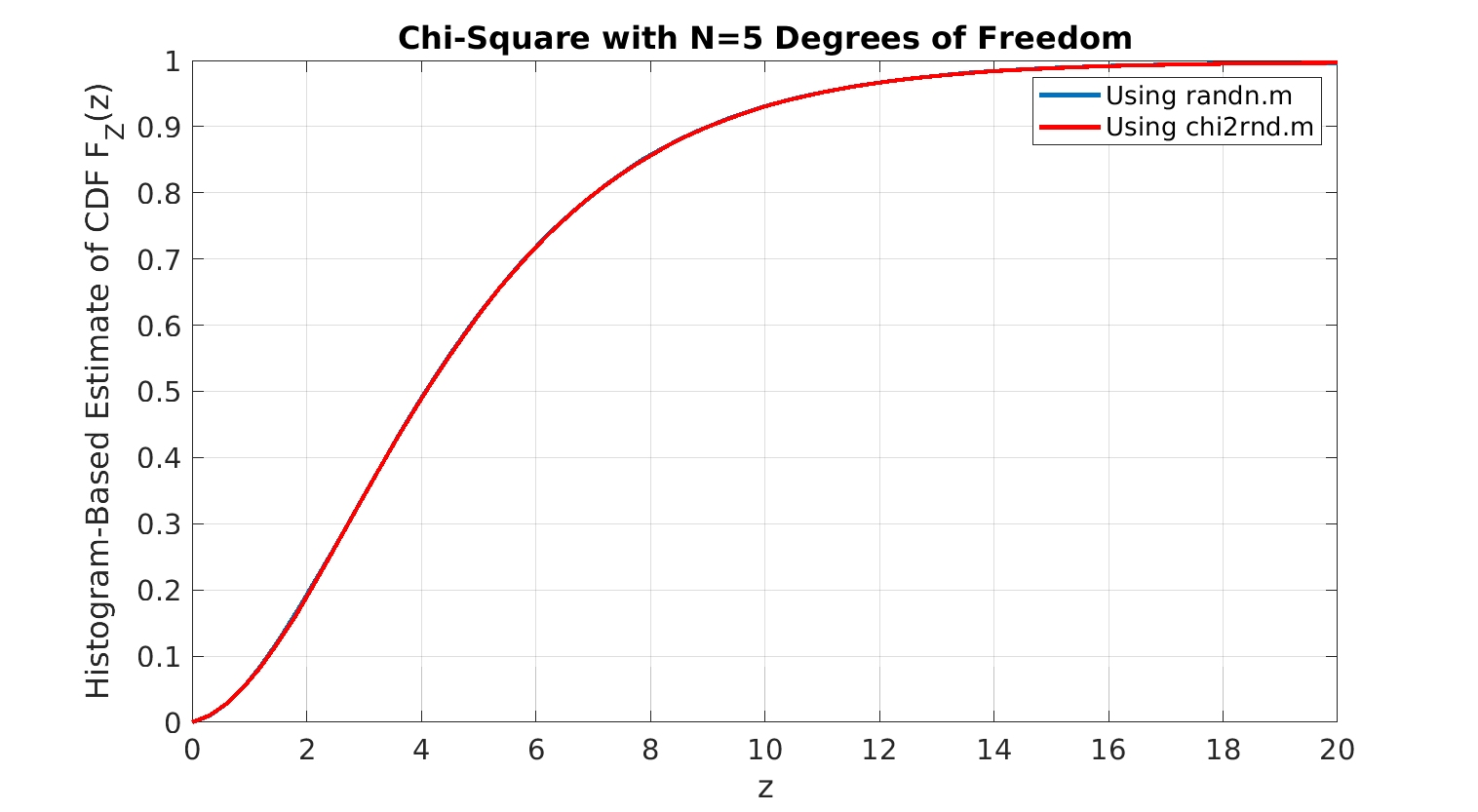Figure 9. Estimated cumulative distribution function for a chi-square random variable with parameter done two ways: with randn.m and with chi2rnd.mFigure 10. Estimated probability density function for a chi-square random variable with parameter done two ways: with randn.m and with chi2rnd.m.

#### Exponential

The exponential distribution has a simple PDF that is characterized by a single parameter we’ll call$a$:$f_X(x) = ae^{-ax}$. This kind of random variable is important in queueing theory, but not so common in signal processing for communication and radar signals and systems. However, when I was a kid I recall seeing published papers on signal processing where the sole difference between one paper and a related one is that the first embedded the signal of interest in Gaussian noise and the second embedded the signal in exponential noise. I could never quite get why that was worth publishing … but then again I don’t get a lot of things.

In queueing theory, events occur at times that are well-modeled by a Poisson random variable (omitted here! better get on that…) and the event interarrival time (times between event times) is an exponential random variable.

Anyway, you can generate instances of the exponential random variable in MATLAB by using exprnd.m. I did that and estimated the PDF and CDF using histcounts.m for a couple different values of$a$; the results are shown in Figures 11 and 12.

#### Log-Normal

A log-normal random variable is one whose logarithm is a Gaussian (normal) random variable. This kind of variable comes up when looking at local (short-time) power measurements as well as in certain kinds of propagation-channel conditions such as shadowing.

You can generate a log-normal random variable in MATLAB in at least two ways: with randn.m and with lognrnd.m. The log-normal distribution will depend on the parameters of the underlying normal random variable, which are just the mean and variance of the normal variable. I generated a large number of log-normal random variables both ways and used histcounts.m to estimate the CDF and PDF, which are shown in Figures 13 and 14. The mean and variance of the normal variable are zero and one, respectively.

To use randn.m, just generate the variable with the desired mean and variance and apply the exponential function exp.m.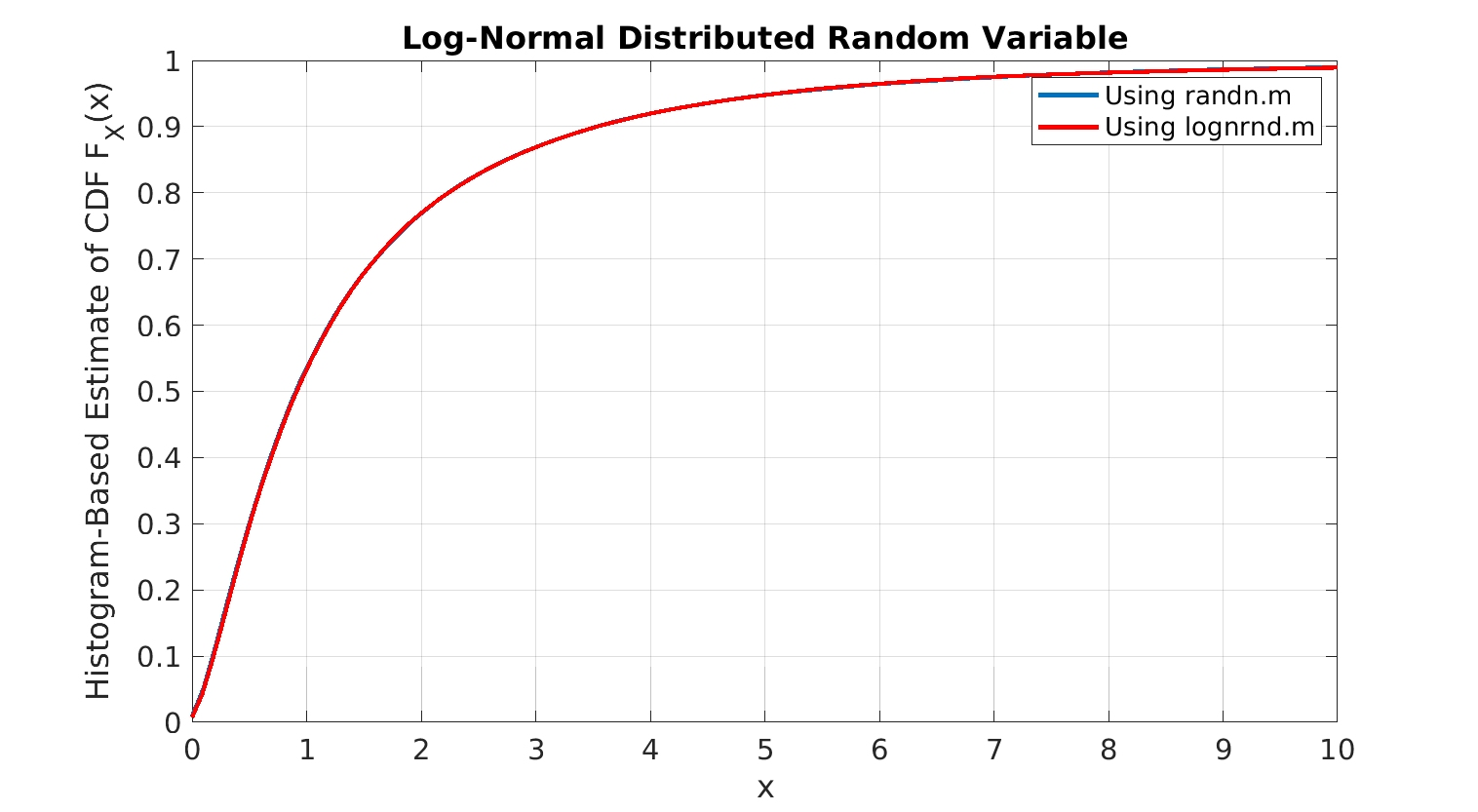Figure 13. Estimated cumulative distribution function for a log-normal random variable. The underlying Gaussian random variable has zero mean and unit variance.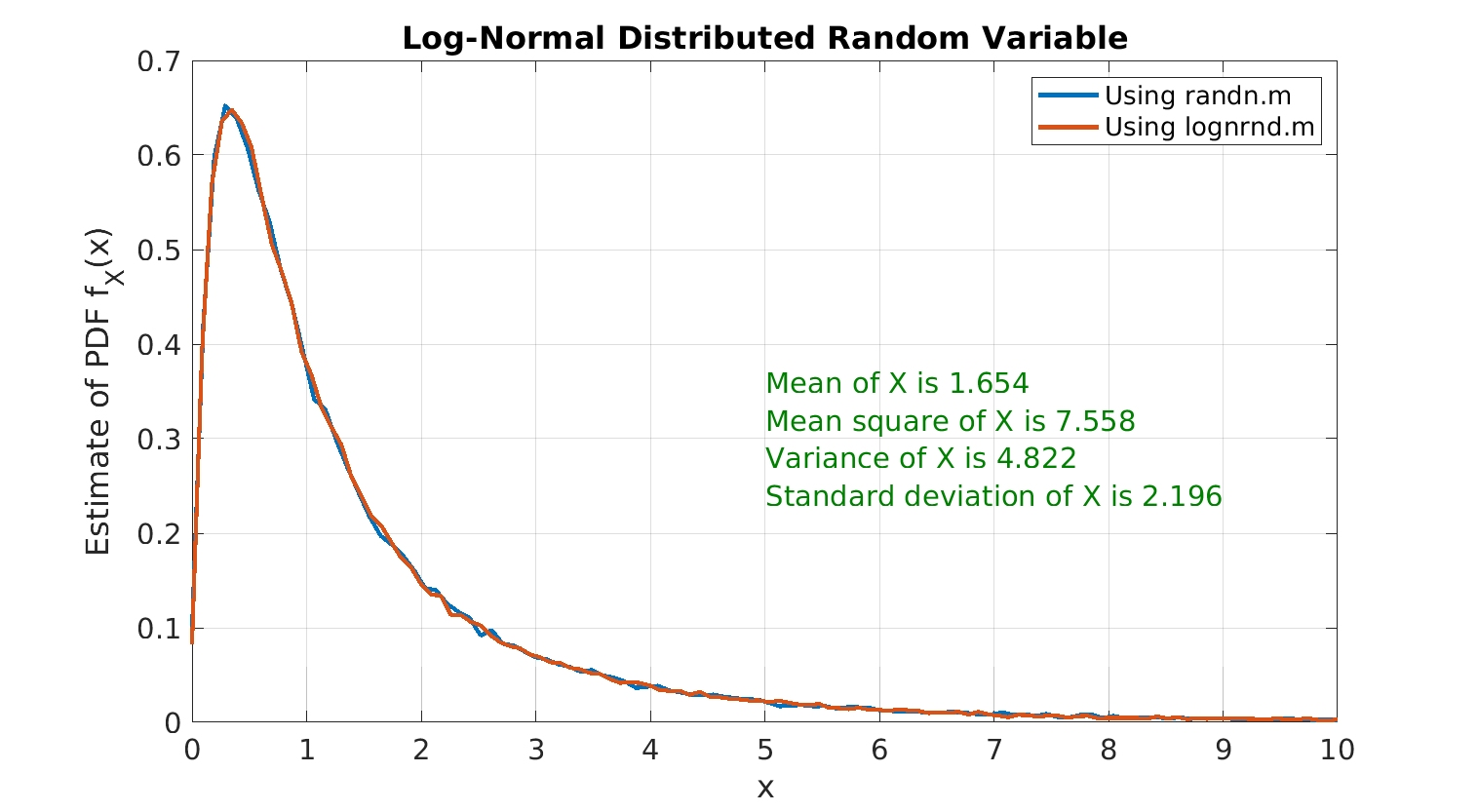Figure 14. Estimated probability density function for a log-normal random variable. The underlying Gaussian random variable has zero mean and unit variance.

### The Bivariate Gaussian Distribution and Correlation Coefficients

Let’s now turn to the case of two random variables. The probabilistic behavior of$N$ random variables is captured in the joint$N$-dimensional probability density and cumulative distribution functions, which we introduced in the random-variable post.

Here our focus is on two jointly Gaussian random variables$X$ and$Y$. By ‘jointly Gaussian’ we simply mean that the two-dimensional PDF is of the standard form for Gaussian random variables.

First we look at the case of two zero-mean uncorrelated jointly Gaussian random variables. We’ll vary their variances and estimate and plot their joint PDF as a surface above a two-dimensional plane, and also we’ll show the aerial view of that surface. The statistics shown in the upper plot of the videos are obtained by using mean.m and var.m in MATLAB.

The variables are generated using MATLAB’s mvnrnd.m, which can be used to generate instances of correlated or uncorrelated Gaussian (normal) random variables for$N ge 1$. The function requires an$N$-dimensional mean-value vector, specifying the mean of each of the$N$ Gaussian random variables, and a correlation matrix, which specifies the variances of the$N$ variables along the main diagonal and the covariances on all the different off-diagonal elements. For example, for our case of$N=2$, we can set the means equal to zero with

mean_vec = [0 0];

and render the variables uncorrelated and with variances of 2 by specifying the matrix

sigma_mat = [2 0; 0 2];

Once the set of instances is generated by mvnrnd.m, we can use histcounts2.m, a two-dimensional version of histcounts.m, to generate the histogram that is normalized to obtain the PDF estimate:

[counts, locsX, locsY] = histcounts2(X(:,1), X(:,2), edgesX, … edgesY, ‘normalization’, ‘pdf’);

surf.m can then be used to generate the plots.

surf(locsX(1:end-1), locsY(1:end-1), counts);

The correlation coefficient can be computed by just applying the formulas we showed in the random-variables post, or by simply using MATLAB’s corrcoef.m, which is what I did here (“Corr coef is …”).

Video 1 shows the case of uncorrelated zero-mean Gaussian random variables for various variances. Video 2 shows the case of uncorrelated zero-mean Gaussian random variables with variances equal to five but with a variety of viewing angles of the surface.

To reveal the effect of correlation on the shape of the joint PDF, I generated sets of zero-mean Gaussian random variables with fixed variances of five, but with a variety of correlation coefficients. This is done by making the off-diagonal elements of the covariance-matrix input to mvnrnd.m equal to the desired correlation coefficient multiplied by the geometric mean of the variables’ variances:

xy = cc*sqrt(var_x*var_y);

sigma_mat = [x_var xy; xy y_var];

Video 3 shows the resulting PDF estimates for correlation coefficients ranging from -1 to 1 in steps of 0.1.

### Convolution and the Central Limit Theorem

Our final topic in this post is the central limit theorem. Recall this theorem says that if you add enough independent random variables together, the resulting random variable has a distribution that is arbitrarily close to a Gaussian, and the distribution of the individual random variables doesn’t matter.

Recall also that when you add together two independent random variables, the probability density function for the sum is the convolution of the two variables’ density functions. So if we add two identically distributed uniform random variables, we can get at the density function of the sum by convolving a rectangle with itself. This is because the density function for all uniform random variables is simply a rectangle.

In this last section, we numerically demonstrate that we can convolve a rectangle with itself and get very close to the Gaussian. Consider the rectangle shown in Figure 15. It has a width of 20 samples and unit area. Convolving it with itself yields the expected triangle in the middle plot. Convolving it with itself twice, or convolving the triangle with the rectangle, yields the smooth function in the bottom plot.

But is that smooth function really approaching a Gaussian?

In Figure 16, I convolve the rectangle with itself 10 times. Using the resulting function as a PDF, I compute its variance and calculate a Gaussian PDF with zero mean and the obtained variance. That Gaussian PDF is then plotted over the top of the 10-times convolved rectangle in the bottom graph of Figure 16. You can see the excellent correspondence. I find it kind of cool that random variables with such non-smooth PDFs (unit steps!) can be added together to get a PDF that is ultimately smooth (infinitely differentiable).Figure 15. A rectangle convolved with itself is a triangle. A rectangle convolved with itself is a smooth pulse-like function.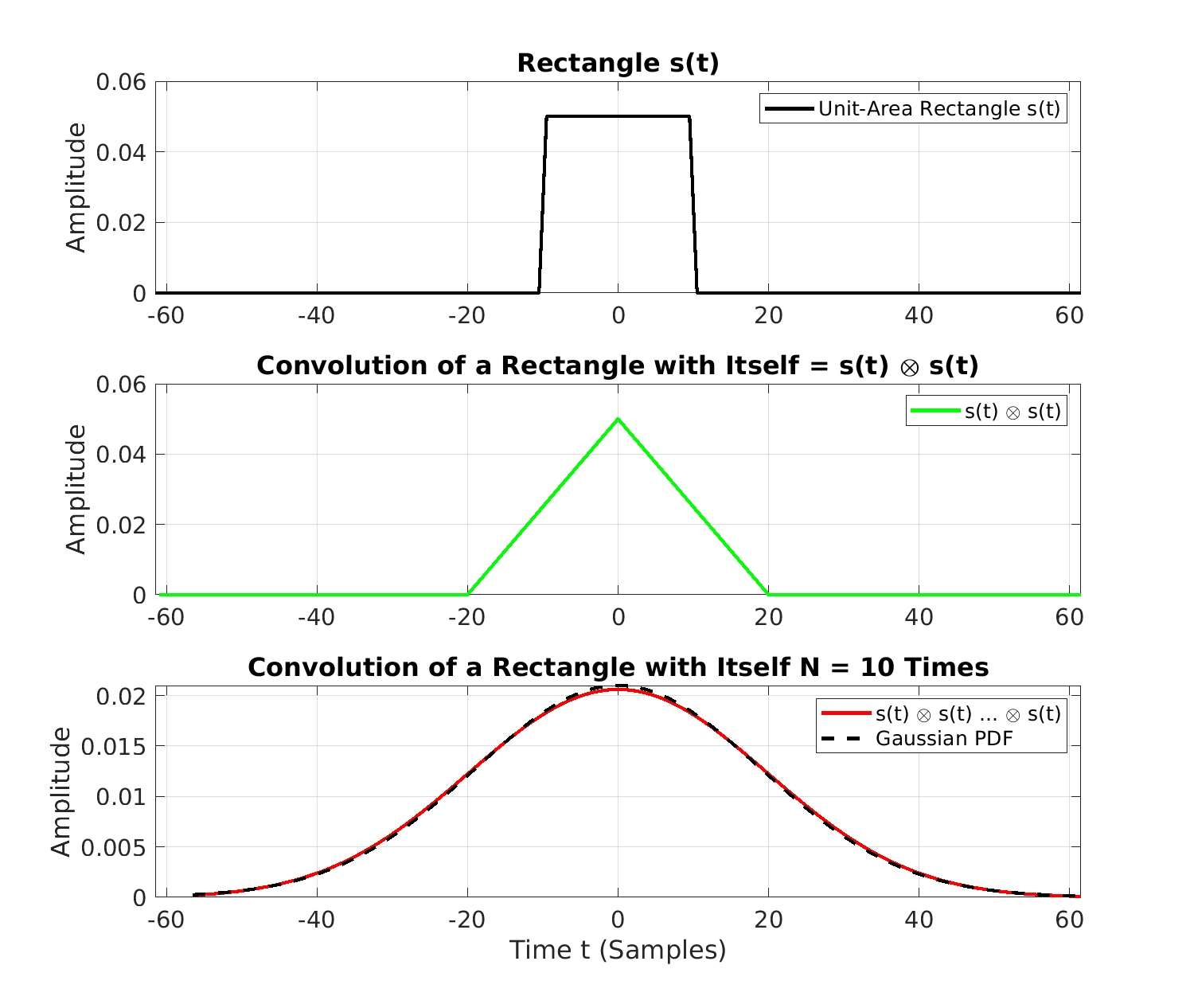Figure 16. A rectangle convolved with itself is a triangle. A rectangle convolved with itself is a smooth pulse-like function. What happens when you convolve a rectangle with itself many times? If the rectangle is interpreted as the probability density function of a uniform random variable, then the density function for the sum of such variables is the convolution of rectangles. Therefore we expect that the convolution of a rectangle with itself a large number of times approaches the Gaussian PDF.

#### Further MATLAB Random-Number-Generation Notes

There are several other random-variable instance generators in MATLAB:Figure 17. Some of the random-variable-related functions available in MATLAB. The function random.m serves as an interface to many of the random-variable generators, but MATLAB says using the generators themselves (*rnd.m) is often faster.

### Significance of Specific Random Variables in CSP

Many random variable types are encountered in the mathematics of communication signals, channels, and systems. Several of those are of little interest to us here because they appear in aspects of the system of little import to the physical-layer signal, which is where we apply CSP. Of those that remain, the most important are the Gaussian (normal) distribution, since it dominates the models of additive noise that characterize all of our RF equipment, and the discrete random variables with uniform distributions, such as the binary$\{+1, -1\}$ equiprobable discrete random variable that models the bits in a BPSK signal, since our digital QAM/PSK signals are highly dependent on these variables.

Several other important random variables are extensively used in communication-channel modeling, such as the Rayleigh, log-normal, and chi-square distributions.

The spectral correlation function is zero-valued for all stationary signals (except for the non-conjugate cycle frequency of zero, which is the power spectrum), which includes the ubiquitous additive white Gaussian noise. The higher-order cumulants and polyspectra, and therefore the higher-order cyclic cumulants and cyclic polyspectra, are zero-valued for all Gaussian signals. These facts render Gaussianity an important aspect of all data that may be subjected to CSP.

Previous SPTK Post: Random Variables Next SPTK Post: Random Processes